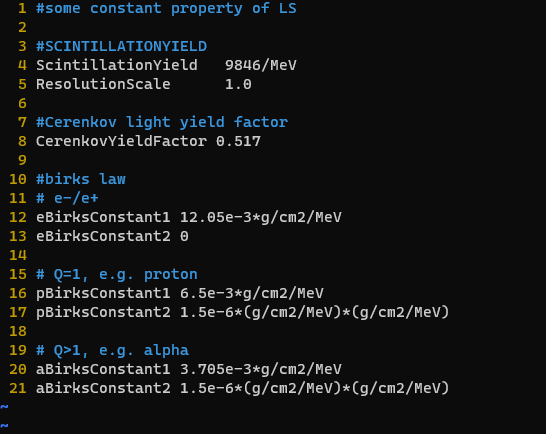# Quenching in mgdraw.f

Dear professors,

I have noticed some relative threads like Mgdraw.f, ENDRAW routing, quenching and I want to ask in detail in several aspects. I attach the default codes as followed.

``````      IF ( LQEMGD ) THEN
IF ( MTRACK .GT. 0 ) THEN
RULLL  = ZERZER
CALL QUENMG ( ICODE, MREG, RULLL, DTQUEN )
WRITE (IODRAW) ( ( SNGL (DTQUEN (I,JBK)), I = 1, MTRACK ),
&                         JBK = 1, NQEMGD )
END IF
END IF
``````
1. Is LQEMGD the flag for quenching and is it true when I activate the USERDUMP with SDUM=UDQUENCH? IF so, where do the birks constants in the USERDUMP go into and how to distinguish different constant of different material as in the card they are denoted simply as birks constant for material 1, 2 and 3?

2. How exactly does subroutine QUENMG work? I can’t find its explanations in the manual. Why does it take in the variable RULLL which is initialized as zero? Why doesn’t it take in DTRACK(I) instead? Because the quenching is directly related to the deposited energy. On the other hand, is DTQUEN the result of quenching energy? More specifically, what do DTQUEN(1,1) and DTQUEN(1,2) mean? Why the same energy deposition point (MTRACK = 1) could have two quenching energies?

3. Provided that I want to assign three groups of birks constants to the same material according to the particle type (as in the picture), how to do that in mgdraw.f?